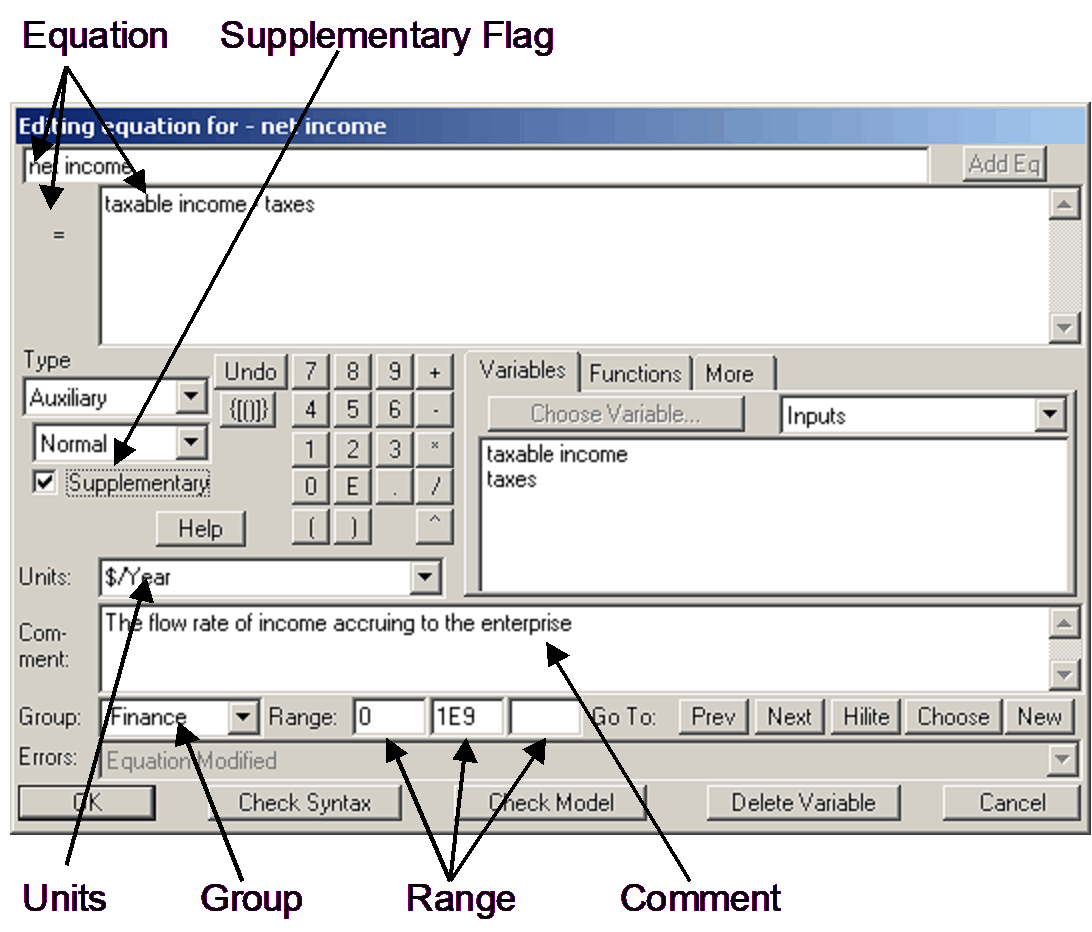# Vensim Help

 Navigation: Reference Guide > The Vensim Modeling Language > Equations Equation Components

The equation for a variable shows how that variable is derived from other variables.  When you specify the equation for a variable you also have an opportunity to specify other important information, including:

 • Units of measure for the variable.
 • A range: minimum and maximum values the variable would be expected to take on along with an increment.
 • A comment, containing description of what the variable is and its importance.
 • The group with which you want the variable associated.
 • A flag to mark the variable as an output only variable (supplementary).

The way you enter this information depends on the tools you use to build your model.  If you use the Equation Editor there are fields for each of these inputs, as can be seen here.Not that the Editor is usually used in conjunction with the Sketch tool which creates the structural relationships between variables.  The Equation Editor then adds in the equation, as well as the other components.

If you are using the Text Edit tool - or storing the model in .mdl format, the different components are delimited using tildes ~ and terminated using a vertical bar |  as shown here:The tilde ~ symbol separates the units from the equation, the comment from the units, and any additional information from the comment.  The vertical bar | signals that a new equation will follow.  Other than the equation, all of the components can be left empty, but you must explicitly recognize this omission by including the delimiters ( ~ and most importantly  |)  after each equation, as in:

net income = taxable income - taxes~~ |

For economy of expression the tilde-tilde-bar ~~| notation will usually be used in the reference guide.  If you are working with the Equation Editor these delimiters will not appear, but the components they delimit will appear in the Equation Edit window as shown above.